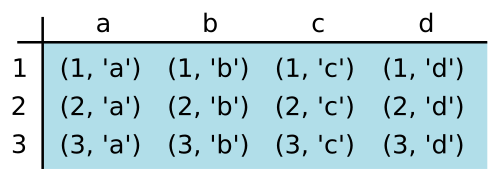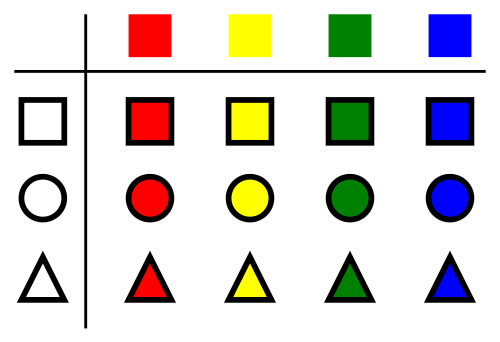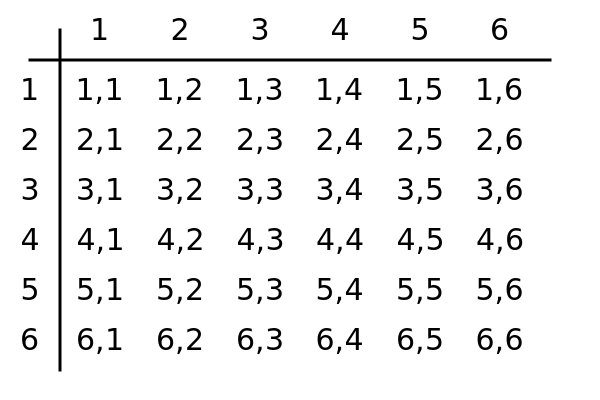# itertools module - cartesian product

By Martin McBride, 2022-08-19
Tags: product itertools python standard library
Categories: python standard libraryThe itertools.product function returns the Cartesian product of two or more iterables.

## What is the Cartesian product?

Suppose we have two sets of values, for example:

• a is the set of values (1, 2, 3).
• b is the set of values ("a", "b", "c", "d").

Suppose we form a pair of values, by taking one value from a, followed by one value from b. What are the possible combinations? One easy way to visualise this is as a two-dimensional table, where the first iterator defines the rows, and the second defines the columns:In this table, the rows represent the values in a and the columns represent the values in b. Using the table is a good way to be sure that we find every possible value. Here are those values:

(1, 'a')(1, 'b'), (1, 'c'), (1, 'd'), (2, 'a'), (2, 'b'), (2, 'c'), (2, 'd'), (3, 'a'), (3, 'b'), (3, 'c'), (3, 'd')


The number of points will always be equal to the length of a multiplied by the length of b.

Another way to visualise the behaviour of product is to consider a nested loop. This loop demonstrates the order of the pairs created in the example above:

for i in a:
for j in b:
print(i, j)


## The itertools product function

The itertools.product function provides a convenient way to generate all the values in a Cartesian product. It can be used like this:

import itertools

a = [1, 2, 3]
b = ["a", "b", "c"]

prod = itertools.product(a, b)

print(prod)
print(list(prod))


In this code, we create two lists, a and b. When we call product it creates an iterator prod. Since this is an iterator, it doesn't create any values until it needs to, so print(prod) just prints an object description.

When we call print(list(prod)) the iterator is realised as a list (see the discussion here), so the print statement displays the value [5, 5, 5].

All code samples can be found on github.

## Examples of Cartesian product

Here are s couple more examples of Cartesian products.

## Shapes and colours

Quite often the product function is used to mix two entirely different sets. For example, let's imagine some new variant of the board draughts (or checkers as it is called in some parts of the world). Imagine this game that uses counters of different shapes and colours - they can be squares, circles or triangles, and can be red, yellow, green or blue.

How many distinct types of pieces are there? Well, there are 3 shapes and 4 colours so that makes 12 variants. Here is a table displaying this:The code to generate these options is similar to the previous code:

shapes = ["square", "circle", "triangle"]
colors = ["red", "yellow", "green", "blue"]

prod = itertools.product(shapes, colors)

print(list(prod))


And here is the result:

[('square', 'red'), ('square', 'yellow'), ('square', 'green'), ('square', 'blue'),
('circle', 'red'), ('circle', 'yellow'), ('circle', 'green'), ('circle', 'blue'),
('triangle', 'red'), ('triangle', 'yellow'), ('triangle', 'green'), ('triangle', 'blue')]


## Throwing two dice

Sometimes both sets in the product might be identical. A good example would be throwing two dice. In that case, the first dice could have a score of 1 to 6, and the second dice could also have a score of 1 to 6.

Since each dice has 6 possible values, there are 36 possible outcomes, as shown in this table:Here is the code to generate this table:

dice1 = range(1, 7)
dice2 = range(1, 7)

prod = itertools.product(dice1, dice2)

print(list(prod))


Here we have used the range function to generate values 1 to 6 (remember that the range function with two arguments counts from the start value up to but not including the end value).

The range function creates a lazy iterable, so we need to create two, one for each dice. If we passed the same iterable in twice, product would attempt to read its values twice, which would fail because a range iterable can only be read once.

This code will create the 36 values shown in the table above:

[(1, 1), (1, 2), (1, 3), (1, 4), (1, 5), (1, 6), (2, 1), ... ]


One thing to notice here is that the order of the numbers is significant. For example the table lists (1, 2) and (2, 1) as separate results.

This is quite important if you are interested in the probability of getting a particular combination. There are 36 possible outcomes of throwing 2 dice so, for example:

• The probability of throwing a two and a one (in any order) is 1 in 18 because there are two possible ways of getting this outcome - either (1, 2) or (2, 1).
• The probability of throwing a double six is 1 in 36 because there is only one possible way of getting this outcome - (6, 6).

## Cartesian product with more than two elements

We can create Cartesian products with more than two sets. For example:

• In the example of draughts pieces, we might have a big and small version of each piece (this sounds like a very complicated game even though it hasn't even been invented yet). So now we would have pieces with any 1 of 3 shapes, 4 colours, and 2 sizes, which makes 24 combinations.
• In the example of dice, we might throw 4 dice at the same time, giving us 6 x 6 x 6 x 6 possibilities

Here is the code in the case of the draughts pieces:

shapes = ["square", "circle", "triangle"]
colors = ["red", "yellow", "green", "blue"]
size = ["big", "small"]

prod = itertools.product(shapes, colors, size)

print(list(prod))


This is the set of results:

[('square', 'red', 'big'), ('square', 'red', 'small'), ('square', 'yellow', 'big'),
('square', 'yellow', 'small'), ('square', 'green', 'big'), ('square', 'green', 'small'),
('square', 'blue', 'big'), ('square', 'blue', 'small'), ('circle', 'red', 'big'),
('circle', 'red', 'small'), ('circle', 'yellow', 'big'), ('circle', 'yellow', 'small'),
('circle', 'green', 'big'), ('circle', 'green', 'small'), ('circle', 'blue', 'big'),
('circle', 'blue', 'small'), ('triangle', 'red', 'big'), ('triangle', 'red', 'small'),
('triangle', 'yellow', 'big'), ('triangle', 'yellow', 'small'), ('triangle', 'green', 'big'),
('triangle', 'green', 'small'), ('triangle', 'blue', 'big'), ('triangle', 'blue', 'small')]


## Difference between Cartesian product and combinations or permutations

How does the Cartesian product compare with combinations and permutations?

Well at first glance it appears to be unrelated. A product takes one item each from two or more sets, whereas combinations and permutations take several items from a single set.

However, when we look at the example of throwing two dice, since each set contains the same elements, the product works in the same way as taking several items from the same set.

In fact, the Cartesian product of the same set is identical to the permutations with replacement.

## Using the repeat parameter

product has an optional repeat parameter that can be used to repeat the input elements. It works like this:

dice = range(1, 7)
prod = itertools.product(dice, repeat=2)


This call will create the product of dice and dice. In other words, it will simulate two dice being thrown, just like we did earlier.

The repeat parameter has an advantage (in addition to avoiding repetition). Recall that previously we had to create two separate dice iterables:

dice1 = range(1, 7)
dice2 = range(1, 7)

prod = itertools.product(dice1, dice2)


This is because a range object can only be read once.

When we use the repeat parameter, the dice sequence is only read once, even though it is used twice.